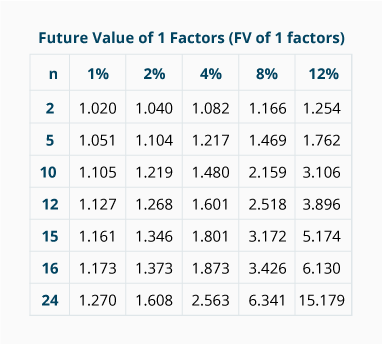# Future Value of a Single Amount (Practice Quiz)

For multiple-choice and true/false questions, simply press or click on what you think is the correct answer. For fill-in-the-blank questions, press or click on the blank space provided.

1. 1. The amount at a later point in time is known as a
__________
value.
2. 2.
__________
interest refers to earning interest on previously earned interest.
3. 3. The amounts contained in a future value of 1 table for various combinations of n and i are referred to as future value
__________
.
4. 4. In the future value of 1 table, n refers to the number of
__________
of time, such as months, quarters, years.
5. 5. If you know the future value of a single deposit, the interest rate, and the number of periods that the interest is compounded, you will be able to calculate the
__________
value by using a future value of 1 table.
6. 6. Assume that you are calculating the future value of a single deposit by using a future value of 1 table. The deposit will be invested in an account earning 12% per year for four years. If the interest will be compounded quarterly, the number of periods (n) will be
__________
and i will be 3%.
7. 7. An unrestricted deposit of \$1,000 grows to a future value of \$5,000 through the compounding of interest. Under the accrual basis of accounting, the \$4,000 of growth should be reported as
__________
.
8. 8.

Under the accrual basis of accounting, the interest earned over a three-year period on a single deposit should be reported on a company's income statement

At The End Of 3 Years
During The 3 Years
9. 9.

Which compounding of an 8% annual interest rate will result in a larger future value?

2% Per Quarter
8% Per Year
No Difference
10. 10. If you know the present value of a single amount, the future value of that amount, and the number of periods that the interest will be compounded, you can calculate the
__________
.
11. 11. When using the future value of 1 table to calculate the future value of a deposit earning 10% per year compounded semiannually, you would use a factor from the column headed
__________
.
12. 12. An account or deposit will earn 12% interest per year for two years. Assuming that you are using the future value of 1 table and the interest is compounded monthly, you will find the factor in the row where n is
__________
periods.
13. 13. An account or deposit will earn 12% interest per year for two years. Assuming that you are using the future value of 1 table and that the interest is compounded monthly, you will find the factor in the column where i is
__________
.
14. Use the following future value of 1 factors for solving the remaining questions:15. 14. A deposit of \$1,000 on January 1, 2023 will have a future value of \$
__________
on December 31, 2027 (or January 1, 2028) if it is invested at 8% per year and the interest is compounded annually.
16. 15. A deposit of \$10,000 on January 1, 2023 will have a future value of \$
__________
on December 31, 2027 (or January 1, 2028) if it is invested at 8% per year and the interest is compounded semiannually.
17. 16. A deposit of \$2,000 on January 1, 2023 will have a future value of \$
__________
on December 31, 2024 (or January 1, 2025) if it is invested at 12% per year and the interest is compounded monthly.
18. 17. A deposit of \$1,000 on January 1, 2023 will have a future value of \$
__________
on December 31, 2026 (or January 1, 2027) if it is invested at 8% per year and the interest is compounded quarterly.
19. 18. The interest rate that is necessary for a deposit of \$1,000 to grow to \$5,474 after 15 years of interest compounded annually is
__________
% per year.
20. 19. The annual interest rate that is necessary for a deposit of \$1,000 to grow to \$1,373 after four years of interest compounded quarterly is
__________
% per year.
21. 20. The number of years required for an investment of \$1,000 to grow to \$2,563 when invested at an annual rate of 8% compounded semiannually is
__________
years.

Want more practice questions?